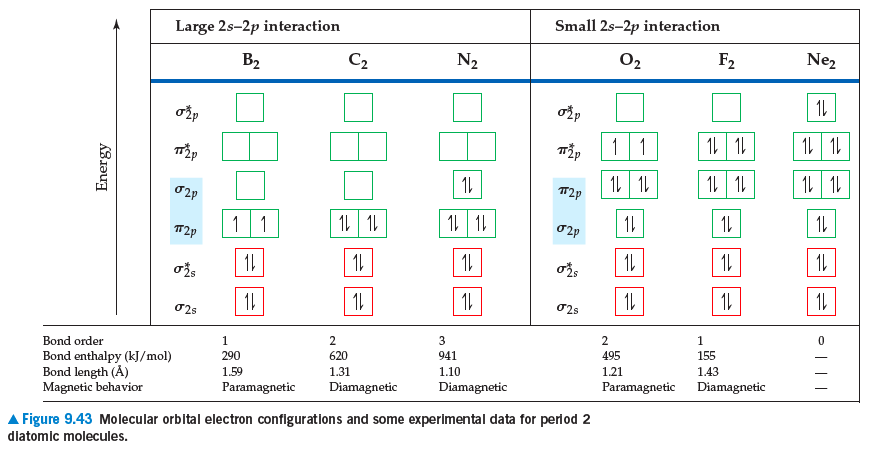# Problem: You may want to reference(Pages 371 - 382)Section 9.8 while completing this problem.If we assume that the energy-level diagrams for homonuclear diatomic molecules shown in Figure 9.42 in the textbook can be applied to heteronuclear diatomic molecules and ions, predict the bond order and magnetic behavior of each: (a) CO+, (b) NO–, (c) OF+, (d) NeF+.

###### FREE Expert Solution
89% (161 ratings)
###### Problem Details

You may want to reference(Pages 371 - 382)Section 9.8 while completing this problem.

If we assume that the energy-level diagrams for homonuclear diatomic molecules shown in Figure 9.42 in the textbook can be applied to heteronuclear diatomic molecules and ions, predict the bond order and magnetic behavior of each: (a) CO+, (b) NO, (c) OF+, (d) NeF+.Frequently Asked Questions

What scientific concept do you need to know in order to solve this problem?

Our tutors have indicated that to solve this problem you will need to apply the MO Theory: Heteronuclear Diatomic Molecules concept. You can view video lessons to learn MO Theory: Heteronuclear Diatomic Molecules. Or if you need more MO Theory: Heteronuclear Diatomic Molecules practice, you can also practice MO Theory: Heteronuclear Diatomic Molecules practice problems.